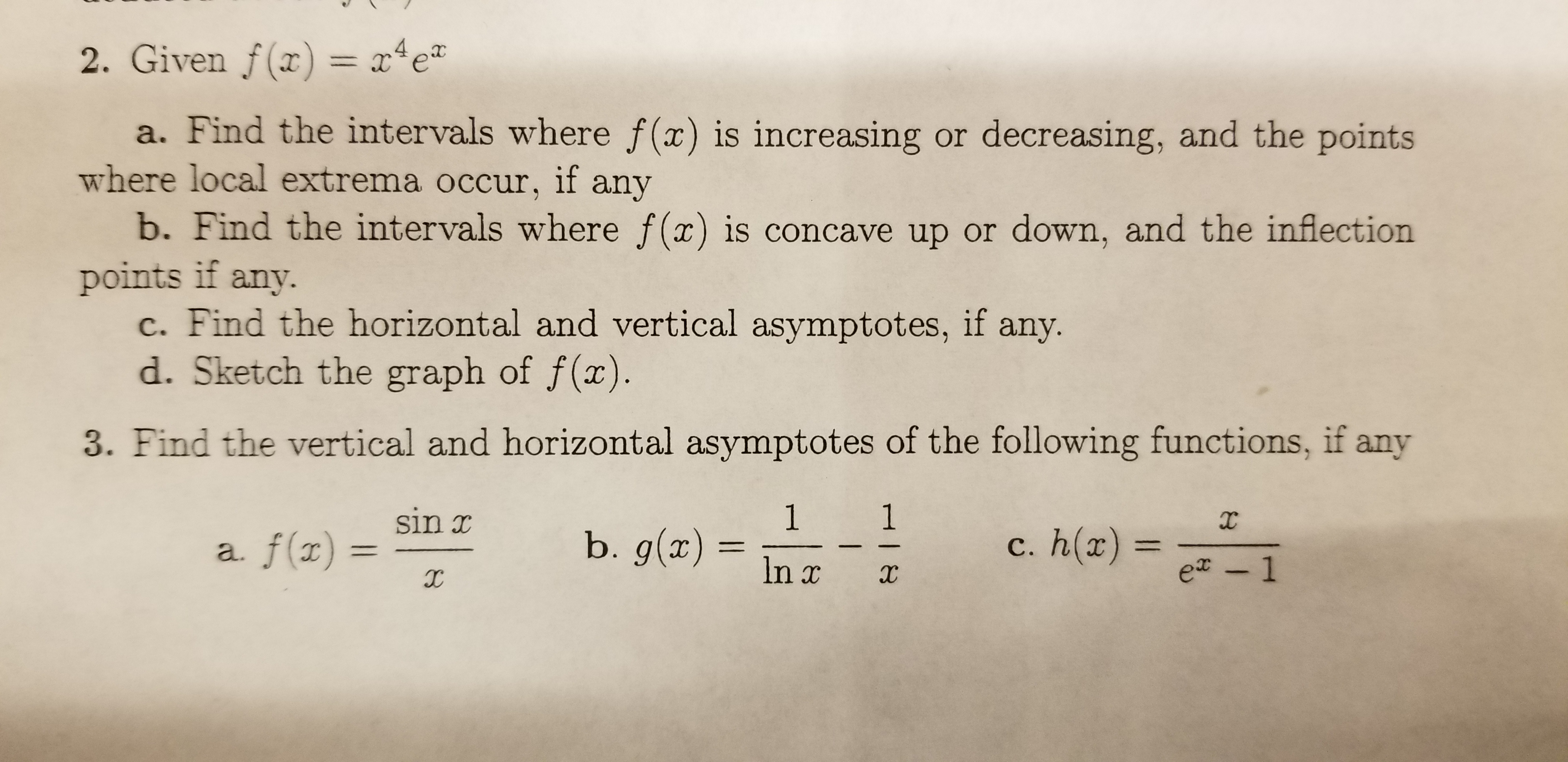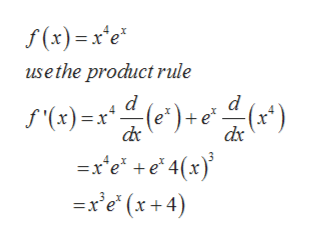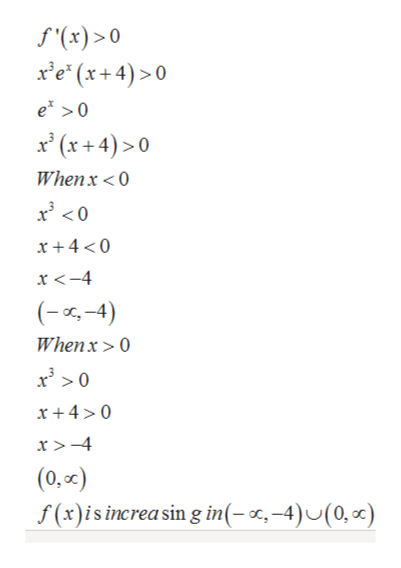# 2. Given f(x) = x4ea. Find the intervals where f(x) is increasing or decreasing, and the pointswhere local extrema occur, if anyb. Find the intervals where f(x) is concave up or down, and the inflectionpoints if any.c. Find the horizontal and vertical asymptotes, ifd. Sketch the graph of f(x).any.3. Find the vertical and horizontal asymptotes of the following functions, if any1b. g(x)1sin xXc. h(x) =a. f(x11e - 1In xHI8

Question
68 views

Question 2 Parts A, B, and Chelp_outlineImage Transcriptionclose2. Given f(x) = x4e a. Find the intervals where f(x) is increasing or decreasing, and the points where local extrema occur, if any b. Find the intervals where f(x) is concave up or down, and the inflection points if any. c. Find the horizontal and vertical asymptotes, if d. Sketch the graph of f(x). any. 3. Find the vertical and horizontal asymptotes of the following functions, if any 1 b. g(x) 1 sin x X c. h(x) = a. f(x 11 e - 1 In x HI8 fullscreen
check_circle

Step 1

For the given function find

1. Interval where the function is increasing or decreasing
2. Interval where the function is concave up or concave down, point of inflection if any
3. Vertical and Horizontal asymptotes if any
Step 2

To find the interval for increasing or decreasing of a function find the first order derivativehelp_outlineImage Transcriptionclose(x)=x*e* use the product rule d (e) + e* =xe +e 4(x) =xe (x+4) d f'(x)=x)() fullscreen
Step 3

F(x) is increasing when ...help_outlineImage Transcriptionclosef(x)> 0 xe (x+4)>0 e >0 x(x+4)>0 Whenx < O x + 4 <0 x <-4 (-4) Whenx >0 x + 40 x >-4 (0,or) f(x)isincreasin g in(-r,-4)U(0, ) fullscreen

### Want to see the full answer?

See Solution

#### Want to see this answer and more?

Solutions are written by subject experts who are available 24/7. Questions are typically answered within 1 hour.*

See Solution
*Response times may vary by subject and question.
Tagged in

### Functions